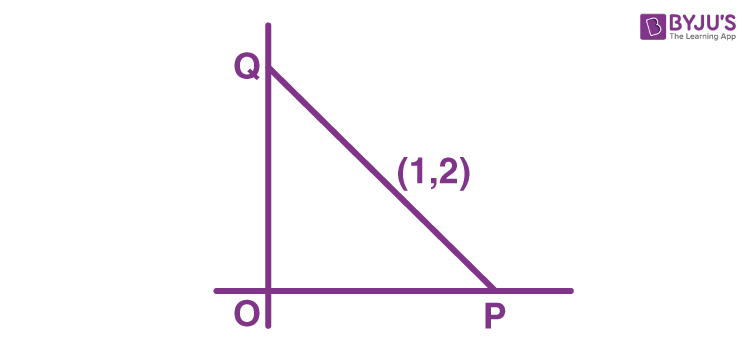# A line is drawn through the point (1,2) to meet the coordinate axes at P and Q such that it forms a AOPQ, where O is the origin, if the area of the AOPQ is least, then the slope of the line PQ is?

Given:

A line through (1,2) meets the coordinate axes at P and O. Also, the area of ΔOPQ is minimum

To find:

The slope of the line PQ

Solution:

Let m be the line PQ, then the equation of PQ is:

y-2=m(x-1)

PQ meets x-axis at P(1-2/m,0) and y-axis at Q(0,2-m)

OP = 1-2/m

OQ = 2-mArea of ΔOPQ = 1/2(OP)(OQ)

=1/2|(1-2/m)(2-m)|

=1/2|4-(m+4/m)|

Let f(m) = 4-{m+4/m)

f'(m) = -1+4/m<sup>2</sup>

f'(m)=0

m<sup>2</sip>=4

m=±2

f(2)=0 and f(-2)=8

We know that the area cannot be zero, therefore, the required value of m is -2.(0)(0)